A common activity in statistics and machine learning is optimization. For instance, finding maximum likelihood and maximum a posteriori estimates require maximizing the likilihood function and posterior distribution respectively. Another example, and the motivating example for this post, is using variational inference to approximate a posterior distribution. Suppose we are interested in a posterior distribution,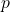, that we cannot compute analytically. We will approximatewith the variational distributionthat is parameterized by the variational parameters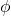. Variational inference then proceeds to minimize the KL divergence fromto,. The dominant assumption in machine learning for the form ofis a product distribution, that is(where we assume there are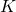variational parameters). It can be shown that minimizingis equivalent to maximizing the evidence lower bound , denoted.

In this post we will consider optimizing the parameters of a probability distribution and will see that using the gradient as in basic calculus can follow suboptimal directions. This can cause slow convergence or convergence to inferior local modes in non-convex problems. Along the way we will introduce some concepts from differential geometry and derive more efficient gradient directions to follow.

For the rest of this post we will assume the setting of variational inference so that the function to optimize isand the components ofare the variational parameters.

Basic calculus tells us that to optimize a continuous function (the objective function) we set the derivative of the function to zero and solve. In all but the simplest cases an analytic solution will not exist and we will need to resort to numerical methods. The simplest numerical optimization algorithm to maximize a function is gradient ascent (descent if one is minimizing a function) which updates the current value of the parameters,, to a new value,, such thatby following the gradient. The update rule is of the formwhereis the gradient vector ofand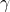determines how for to move along the gradient. The gradient vector points in the direction that the function increases most quickly, where changes in the function are measured with respect to Euclidean distance. In other words, the gradient indicates the direction such that the smallest change in parameter values results in the largest change in function value, where changes in the parameters and function values are measured by the Euclidean distance. If the Euclidean distance between the variables being optimized (the variational parameters in our running example) is not a good measure of variation in the objective function then gradient ascent will move suboptimaly through the parameter values.

The Euclidean distance between parameter values of distributions turns out to not be a good measure of the variation between the two distributions. As a simple example  consider the two distributionsandas well as the two distributionsand. The first pair of distributions are essentially the same whereas the second two do not share any significant support. Yet, the Euclidean distance between the parameters in the first pair is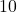, while in the second the distance is only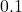. The reason for this is that probability distributions do not naturally live in Euclidean space but rather on a manifold (a statistical manifold to be precise). There are better ways of defining the distance between distributions, one of the simplest being the symmetrized Kullback-Leibler divergencewhich will arise later.

To understand how to improve the gradient ascent algorithm when optimizing the parameters of probability distributions we must understand a bit of differential geometry. Assume thatwhereis a parameter space of interest. Whenis Euclidean space with an orthonormal basis the squared distance between two pointsandis given by the usual Euclidean inner productWhenis a manifold the previous distance is given by the bilinear formThe matrixis called the Riemannian metric tensor and is an inner product on the tangent space of the manifoldat the point. This means that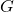allows us to define a norm, and thus distances on the manifold (the tangent space at a point can be thought of as the space of directional derivatives at the point). Note thatdepends on the location, a consequence of the fact thatis defined with respect to the tangent space atand so is location dependent.

As a concrete example, imagine two people standing on two different mountain tops. If one of the people is Superman (or anybody else who can fly) then the distance they would fly directly to the other person is the Euclidean distance (in). If both people were normal and needed to walk on the surface of the Earth the Riemannian metric tensor tells us what this distance is from the Euclidean distance. Don’t take this illustration too seriously since technically all of this needs to take place in a differential patch (a small rectangle whose side lengths go to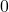). Intuitively, the Riemannian metric tensor describes how the geometry of a manifold affects a differential patch,, at the point. The length of a line between two points onis the distance between them. The Riemannian metric tensor either stretches or shrinks that line and the resulting length is the distance between the two points on the manifold.

In Euclidean space with an orthonormal basisis simply the identity matrix. Whenis a space of parameters of probability distributions and the symmetrized KL divergence is used to measure the distance between distributions thenturns out to be the Fisher information matrix. This arises from placing an inner product on the statistical manifold of log-probability densities .

As stated above, the gradient vector of a functionpoints in the direction of steepest ascent. We can formally write this as maximizesuch thatforsmall. To solve this optimization problem we setfor some vector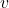. We can then rewrite the optimization problem as maximizingunder the constraint that, that ismust be unit length under the inner product defined byand so we are searching for the direction that causes the function to increase the most where distances arise from the inner product defined by. We can rewrite this constrained optimization problem as an unconstrained problem using Lagrange mulitpliersand after taking the derivative with respect toand setting it to zero we obtainand so. We can then solve for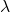using the unit-vector constraint on. We see that whenis Euclidean space andis the identity matrix then the usual gradient vector of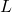is the solution. Whenis non-trivial the vectoris called the natural gradient and as we have just seen is the direction thatincreases most quickly when the parameter space is a manifold with Riemannian metric. This tells us that a more efficient variational inference algorithm is to follow the natural gradient of the variational parameters, where the Riemannian metric tensor is just the Fisher information matrix of the variational distribution.

The derivations in this paper followed that in  which goes on to show what the natural gradient looks like for neural networks and other models and proves that gradient descent with the natural gradient is efficient in a certain sense. The application of differential geometry to statistics is a deep subject. See the book “Differential Geometry and Statistics”  for a nice introduction. Perhaps in a future post we can delve into why the Fisher information matrix appears as the Riemannian metric tensor for statistical manifolds.

References:
 Amari, S., Natural Gradient Works Efficiently in Learning. In Neural Computation, Vol. 10, No. 2, 1998.
 Hoffman, M., Blei, D. M., Wang, C., Paisley, J., Stochastic Variational Inference.  arXiv: 1206.7051.
 Murray, M.K., Rice, J.W., Differential Geometry and Statistics. Monographs on Statistics and Applied Probability, No. 48, 1993.